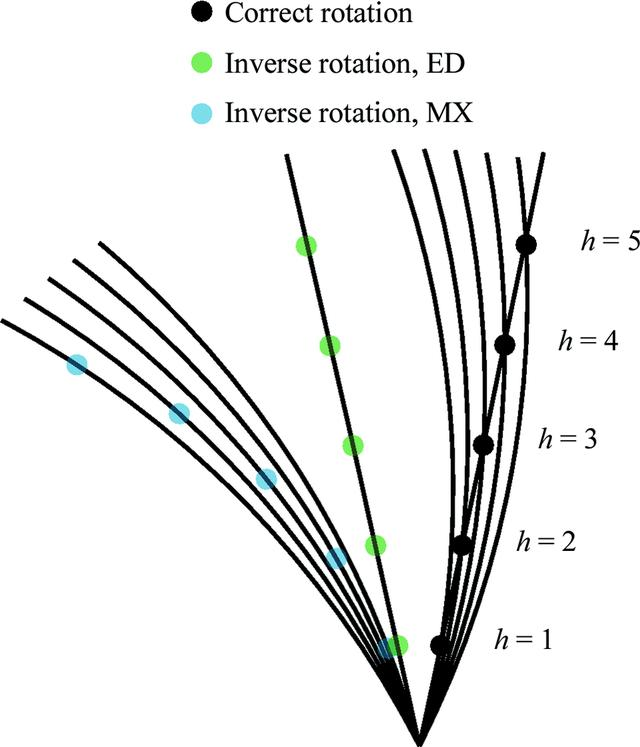disable zoom     view article Figure 3 Five reciprocal-lattice points are shown (in black and labelled) along the a∗ axis for a crystal with unit-cell dimension a = 10 Å. Arcs representing the surface of the Ewald sphere with a typical X-ray wavelength of λ = 1.0332 Å intersect these points at rotation angles between 15.0° for h = 1 and 27.0° for h = 5, where rotations are assumed to be clockwise from vertical in the plane of the figure. If the modelled rotation axis is inverted then φ centroids of observed spots would be mapped onto Ewald spheres rotated between −15.0 and −27.0°, resulting in a distinct curvature to the reconstructed reciprocal lattice (points shown in blue). In the case of electron diffraction at λ = 0.02508 Å the spots are observed almost simultaneously at rotation angles between 12.1 and 12.4°. For clarity a single Ewald arc is shown for h = 3. If the assumed axis is inverted then φ centroids between −12.1 and −12.4° still result in almost a straight line (points shown in green). It is therefore difficult to determine the correct direction of rotation from the appearance of the reconstructed reciprocal lattice alone.STRUCTURALBIOLOGY
ISSN: 2059-7983
Volume 74| Part 6| June 2018| Pages 506-518